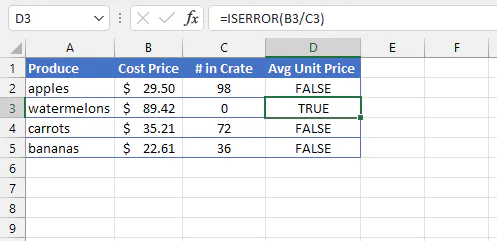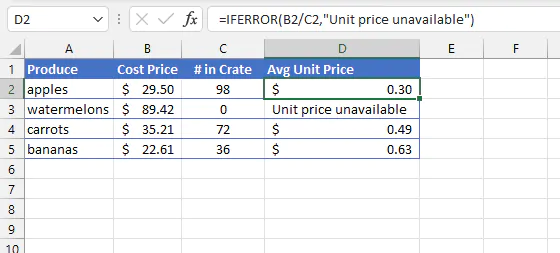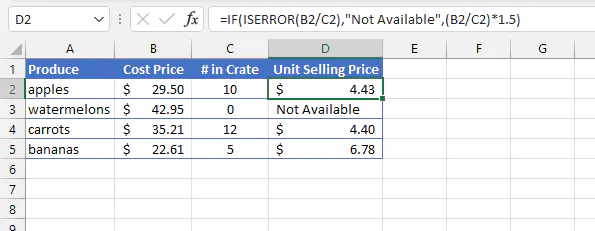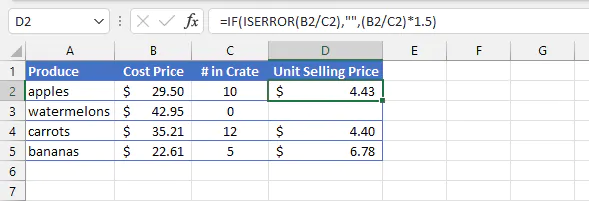# How to Use the Excel ISERROR FunctionClaudia Buckley#### Join the Excel conversation on Slack

Ask a question or join the conversation for all things Excel on our Slack channel.

The Excel ISERROR function is found in the Information category of the Excel Functions Library. Like all other “IS” functions, it gives a specific piece of information about the expression being evaluated. In this case, ISERROR answers the question: will this input result in an error?

An error is defined as any of the following Excel responses:

#N/A, #VALUE!, #REF!, #DIV/0!, #NUM!, #NAME?, #NULL!, #CALC!, or #SPILL!

At some point in your Excel usage, you’ve likely come across at least some of these messages. They don’t mean Excel isn’t working; quite the opposite - they mean you’ve entered something Excel isn’t expecting, or which isn’t a valid input. Each of the above Excel error responses gives a particular clue into what went wrong.

ISERROR is sometimes used to test for the presence of any of these errors by returning a value of TRUE.

## Syntax

The syntax, or format, of ISERROR is:

ISERROR(value)

Value is the cell reference, logical expression, value, or calculation to be evaluated.

The return value of ISERROR is either TRUE or FALSE.

## Basic example

A simple example of ISERROR at work is shown as follows:

``=ISERROR(B3/C3)``The ISERROR formula in cell D3 returns a value of TRUE because the B3/C3 operation would have resulted in a #DIV/0! error. (This is because division by zero is considered mathematically impossible.) The other cells in Column D display FALSE because they would have returned valid mathematical results.

## Purpose

This function can be useful simply for identifying operations or formulas that will result in an error. More often though, this piece of information is plugged into another formula, where TRUE vs. FALSE lead to different decisions.

## ISERROR vs. IFERROR

There's another function that's closely related to ISERROR, called IFERROR. The key difference between IFERROR and ISERROR is this:

• ISERROR is considered informational, and simply returns TRUE or FALSE.
• IFERROR is a logical (decision-making) function. If an error is found, you will state a value to be displayed or alternative calculation to be performed. If no error is found, the result of the original calculation is automatically returned.

IFERROR has two arguments, and they are both required. The syntax of IFERROR is

``IFERROR(value, value_if_error).``

Below is a scenario of how IFERROR is typically used.

``=IFERROR(B2/C2,"Unit price unavailable")``Notice that IFERROR assumes that if there is no error, the result of the value argument should be returned.

## Using IF and ISERROR together

Prior to Excel 2007, ISERROR was used in combination with the IF function to achieve a result similar to what IFERROR accomplishes today. However, this option may still be used when we want a response other than the result of the original calculation to be returned.

The IF function is used to return one value if a statement is TRUE, and another value if the statement is FALSE. The syntax of the IF function is:

``IF(logical_test,[value_if_true],[value_if_false])``

The first argument, logical_test, is required. Of the other two arguments, at least one is required. When nested with ISERROR, the value_if_false argument of the IF function can be used to return an alternative response if a calculation results in an error. An example is shown below:

``=IF(ISERROR(B2/C2),"Not available",(B2/C2)*1.5)``The inner portion of this nested formula, ISERROR(B2/C2), checks if the calculation will result in any of the error messages stated earlier. If so, the response “Not Available” is returned. Notice that the text value is within double quotation marks.

If the operation does not result in an error, the value_if_false is set to an alternative calculation, (B2/C2)*1.5 to work out the unit selling price.

This combination can be useful for communicating a friendly message to end-users. If you want cells with error messages to display a blank cell, the value_if_true should be set to two double quotes, such as below.The above format is helpful when we anticipate certain types of errors and we do not want them to detract from valid mathematical responses.

There are other Excel functions that are similar to ISERROR, for example, ISERR and ISNA. ISERR checks for errors excluding the #N/A error, and ISNA only checks for #N/A errors. These are also informational functions, meaning that they return either TRUE or FALSE.

Check out our extensive resource library to learn how to use other Excel functions!

Become a certified Excel ninja with GoSkills bite-sized courses#### Join the Excel conversation on Slack

Ask a question or join the conversation for all things Excel on our Slack channel.Claudia Buckley

Claudia is a project manager and business skills instructor at GoSkills. In her spare time, she reads mystery novels and does genealogy research.